# Biot-Savart Law¶

The Biot-Savart Law derives the magnetic field B due to a current: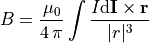Here,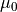is the magnetic constant,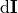is the infinitesimal length and direction of a section of infinitesimally thin wire current we are integrating over, I is the amount of current in that section, and r is the displacement vector from the section of wire current to the point where B is measured.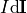may also be replaced with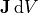if a current density J occupies a volume element dV.

## SIMION Specific¶

SIMION 8.0.3 can compute magnetic fields from wire currents using the Biot-Savart law using the simionx.MField - Biot-Savart magetic field calculations library. In SIMION 8.1.0.19, these wires can also be drawn on the SIMION View screen. Examples of this include

A thick wire can be approximated with many thin wires. See Example(helmholtz_coil) (thick_coil sub-example) added 2018-03-08.